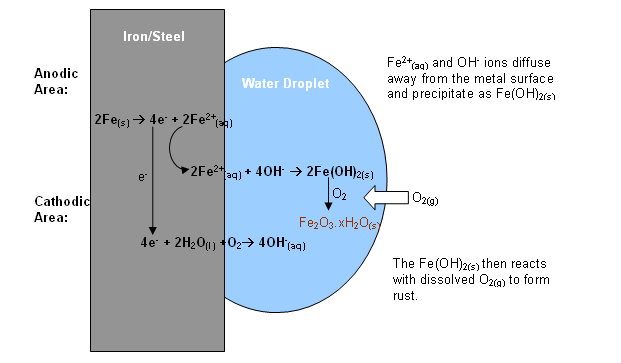# What is the equation when iron reacts with oxygen to produce iron(III) oxide ?

Jan 13, 2015

$F e \to F {e}^{3 +} + 3 {e}^{-}$ iron is oxidised: it's losing electrons
${O}_{2} + 4 {e}^{-} \to 2 {O}^{2 -}$ oxygen is reduced, it's gaining electrons

Balance for the electrons.
The smallest common product of 3 and 4 is 12.
So we get:
$4 F e \to 4 F {e}^{3 +} + 12 {e}^{-}$
$3 {O}_{2} + 12 {e}^{-} \to 6 {O}^{2 -}$

$4 F e + 3 {O}_{2} + 12 {e}^{-} \to 4 F {e}^{3 +} + 12 {e}^{-} + 6 {O}^{2 -}$

The electrons balance so they cancel out:
$4 F e + 3 {O}_{2} \to 4 F {e}^{3 +} + 6 {O}^{2 -}$

Since $F e$ and $O$ can combine in a ratio of 2:3
$4 F e + 3 {O}_{2} \to 4 F {e}^{3 +} + 6 {O}^{2 -} \to 2 F {e}_{2} {O}_{3}$

Extra :
Pure iron won't rust, because the oxide layer would form a protective skin (as aluminium does). It needs some impurities (mainly carbon) and, apart from the oxygen, some moisture -- even carbondioxide 'helps'. In that case iron-hydroxy-oxides and -carbonates are formed, and these flake, no longer protecting the iron underneath.

Jan 13, 2015

Meneer has written an excellent answer so I will add some information which he has referred to in his extra paragraph.

Rusting is fastest when iron and steel is exposed to a combination of air and water and is also affected by impurities in the iron and the presence of other electrolytes.

Rusting is an electrochemical process. Cells are set up in the metal surface where different areas act as sites of oxidation and reduction.

The two half - cells are:

$F {e}_{\left(a q\right)}^{2 +} + 2 e \rightarrow F {e}_{\left(s\right)} {E}^{0} = - 1.44 V$

$\frac{1}{2} {O}_{2 \left(g\right)} + {H}_{2} {O}_{\left(l\right)} + 2 e \rightarrow 2 O {H}_{\left(a q\right)}^{-} {E}^{0} = + 0.40 V$

The most positive half - cell is the one which will take in electrons so you can see that ${O}_{2}$/${H}_{2} O$ will oxidise $F e$ to $F {e}^{2 +}$.

The diagram shows what happens when a drop of water is left in contact with iron or steel:At the edge of the drop where the concentration of dissolved oxygen is higher, oxygen is reduced to hydroxide ions:

${O}_{2 \left(g\right)} + 2 {H}_{2} {O}_{\left(l\right)} + 4 e \rightarrow 4 O {H}_{\left(a q\right)}^{-}$

The electrons needed to do this flow in from the oxidation of iron at the centre of the drop. (Known as "pitting"). Here the $F {e}^{2 +}$ ions pass into solution:

$2 F {e}_{\left(s\right)} \rightarrow 2 F {e}_{\left(a q\right)}^{2 +} + 4 e$

As the $F {e}^{2 +}$ and $O {H}^{-}$ ions diffuse away from the surface they do not form a protective layer but a precipitate of iron(II) hydroxide which "flakes" away. This is then oxidised further by oxygen:

$F e {\left(O H\right)}_{2 \left(s\right)} \rightarrow F {e}_{2} {O}_{3.} x {H}_{2} {O}_{\left(s\right)}$